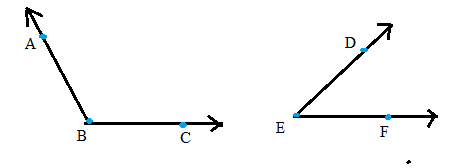Chapter A.3, Problem 3EElementary Geometry For College St...

7th Edition
Alexander + 2 others
ISBN: 9781337614085

Solutions

Chapter
SectionElementary Geometry For College St...

7th Edition
Alexander + 2 others
ISBN: 9781337614085
Textbook Problem

If angles ABC and DEF in the accompanying drawing were measured with a protractor, what does intuition tell you about the degree measures of these angles?To determine

To write:

The intuition about the following degree measures of these angles,Explanation

Consider the following degree measures of these angles,

The angles mABC is an obtuse angle since mABC>90 and mDEF is an acute angles since mDEF<90.

That is mABC larger than mDEF

Still sussing out bartleby?

Check out a sample textbook solution.

See a sample solution

The Solution to Your Study Problems

Bartleby provides explanations to thousands of textbook problems written by our experts, many with advanced degrees!

Get Started

Define the terms population and sample, and explain the role of each in a research study.

Essentials of Statistics for The Behavioral Sciences (MindTap Course List)

Find dy/dx by implicit differentiation. 16. xy=x2+y2

Single Variable Calculus: Early Transcendentals, Volume I

If f(x)=3+x+ex, find (f1)(4).

Calculus (MindTap Course List)

True or False: A straight (simple) substitution may be used to evaluate 2+lnxxdx.

Study Guide for Stewart's Single Variable Calculus: Early Transcendentals, 8th

True or False: is a convergent series.

Study Guide for Stewart's Multivariable Calculus, 8th